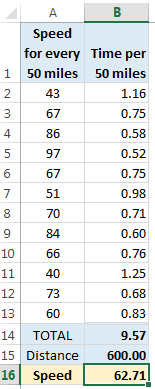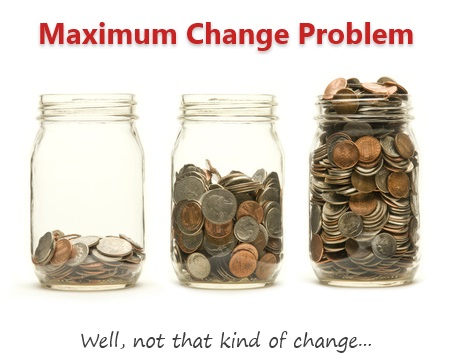# All articles in 'Formula Forensics' Category

## A clever technique to simplify your long, nested IF formulas

Published on Apr 11, 2023 in Formula Forensics, Learn ExcelRecently I used an elegant and smart technique to simplify lengthy nested IF formulas and made them 80% shorter. In this article, let me explain the process and share the results.

Continue »

## Formula Forensics 043: Rankifs or Conditional Rank

Published on Oct 19, 2017 in Formula Forensics, Huis, Posts by HuiLearn how to develop a Conditional Rank or Rankifs formula in Excel

Continue »

## Formula Forensics 042: Reverse Text – A Formulaic Solution

Published on Jan 4, 2017 in Formula Forensics, Huis, Posts by HuiUse the new Textjoin() function to reverse a string of characters suing a formulaic solution.

Continue »

## Formula Forensics No. 041 – Convert a Roman Numeral to a Number

Published on Sep 14, 2016 in Excel Howtos, Formula Forensics, Huis, Posts by HuiLearn how to convert a Roman Numeral to a Number using this nifty formula. No VBA required.

Continue »

## Formula Forensics 040 – Apportioning Sales by Criteria

Published on Feb 15, 2016 in Excel Howtos, Formula Forensics, Huis, Posts by HuiLets look at how to apportion sales according to multiple criteria

Continue »

## Formula Forensics No. 039 – Find the Cell Address for a value (2D & 3D Reverse Lookup)

Published on Oct 15, 2015 in Formula Forensics, Huis, Posts by HuiFind the Cell Address for a value from a 2D or 3D Range
(2D & 3D Reverse Lookup)

Continue »

## Formula Forensics No. 038 – Find Which Worksheet a Max or Min Value is located on

Published on Oct 14, 2015 in Formula Forensics, Huis, Posts by HuiLearn how to find which worksheet a max or min value occurs on using this neat formula

Continue »

## Formula Forensics No. 003b – Lukes Reward – Part II

Published on Nov 10, 2014 in Formula Forensics, Huis, Posts by HuiA Formula based solution to extract records from a table that match a number of criteria.

Continue »

## What is the average speed of this road trip? [Solution & Discussion]

Published on Aug 18, 2014 in Excel Howtos, Formula ForensicsLast week, we had an interesting homework problem – What is the average speed of this road trip?

We received more than 150 answers. But to my surprise, 57 of them are wrong. So today, lets learn how to calculate the average speed correct way.

Continue »

## Formula Forensics No. 037 – How to Count and Sum Filtered Tables

Published on Jul 23, 2014 in Formula Forensics, Huis, Posts by HuiHow to Count and Sum data from Filtered Tables

Continue »

## Calculating Maximum Change [solutions & discussion]

Published on Mar 26, 2014 in Excel Howtos, Formula ForensicsLast Friday, we had a fun little Excel challenge – Calculate Maximum Change. More than 170 people commented and shared their solutions to this problem.

And the best part?

The best part is the variety of solutions & thinking displayed by our community. So if you are one of those 170, puff your chest & pat yourself on the back. Go ahead, I will wait.

Today, lets take a look at some of these awesome formulas and understand how they work. Read on and watch the video you below to gain few awesomeness pounds.

Continue »

## Formula Forensics-No. 036: Calculating Costs that Vary by Year and Age

Published on Nov 26, 2013 in Excel Howtos, Formula Forensics, Posts by Sajan

In this episode of Formula Forensics, lets talk about how to build a model where costs vary per year and age. In this example, we are talking about a plantation project. The costs & yields of plants vary by the year they are planted in and the age of plants. In this case, how do we calculate the total costs or returns with formulas?Continue »

## Formula Forensics No. 035 Average the last 3 values greater than 0

Published on Oct 3, 2013 in Formula Forensics, Posts by HuiLearn a technique to Average the last 3 values from a range where the values are greater than 0

Continue »

## Formula Forensics No. 34. Extract words from a cell, where they occur in a list of words.

Published on Sep 12, 2013 in Formula Forensics, Huis, Posts by HuiUse Excel to extract matching words from a list of words from a cell containing a sentence into individual cells in the order they exist

Continue »

## Formula Forensics No. 033 – Interpolation

Published on Feb 8, 2013 in Formula Forensics, Huis, Posts by HuiToday, Formula Forensics examines 3 techniques to determine an interpolated value along a curve.

Continue »

### Get FREE Excel & Power-BI Newsletter

One email per week with Excel and Power BI goodness. Join 100,000+ others and get it free.